geom_braid() is an extension of geom_ribbon() that uses stat_braid() to correctly fill the area between two alternating series (lines or steps) with two different colors.

## Usage

geom_braid(
mapping = NULL,
data = NULL,
position = "identity",
...,
method = NULL,
na.rm = NA,
show.legend = NA,
inherit.aes = TRUE
)

stat_braid(
mapping = NULL,
data = NULL,
geom = "braid",
position = "identity",
...,
method = NULL,
na.rm = NA,
show.legend = NA,
inherit.aes = TRUE
)

## Arguments

mapping

Set of aesthetic mappings created by aes() or aes_(). If specified and inherit.aes = TRUE (the default), it is combined with the default mapping at the top level of the plot. You must supply mapping if there is no plot mapping.

data

The data to be displayed in this layer. There are three options:

If NULL, the default, the data is inherited from the plot data as specified in the call to ggplot().

A data.frame, or other object, will override the plot data. All objects will be fortified to produce a data frame. See fortify() for which variables will be created.

A function will be called with a single argument, the plot data. The return value must be a data.frame, and will be used as the layer data. A function can be created from a formula (e.g. ~ head(.x, 10)).

position

Position adjustment, either as a string, or the result of a call to a position adjustment function.

...

Other arguments passed on to layer(). These are often aesthetics, used to set an aesthetic to a fixed value, like colour = "red" or size = 3. They may also be parameters to the paired geom/stat.

method

Intersection and imputation method to use to braid the ribbon, accepts NULL, "line", or "step". For method = NULL, the default, print a message to the console and use method = "line". For method = "line", silently braid the ribbon with two series drawn by geom_line() or geom_path(). For method = "step", silently braid the ribbon with two series drawn by geom_step().

na.rm

If NA, the default, missing values are imputed by method. If FALSE, missing values are kept and appear as gaps in the ribbon. If TRUE, missing values are removed.

show.legend

logical. Should this layer be included in the legends? NA, the default, includes if any aesthetics are mapped. FALSE never includes, and TRUE always includes. It can also be a named logical vector to finely select the aesthetics to display.

inherit.aes

If FALSE, overrides the default aesthetics, rather than combining with them. This is most useful for helper functions that define both data and aesthetics and shouldn't inherit behaviour from the default plot specification, e.g. borders().

geom

Override the default connection with geom_braid().

## Value

A ggplot2 layer that can be added to a plot created with ggplot().

## Examples

library(ggplot2)

# To demonstrate the features of geom_braid() we'll use a subset of the
# txhousing dataset from ggplot2.

tx_long <- with(txhousing, txhousing[city %in% c("Dallas", "Austin"), ])
tx_long <- with(tx_long, tx_long[date >= 2008, ])
tx_long <- subset(tx_long, select = c(date, city, inventory))

tx_wide <- data.frame(
date = with(tx_long, date[city == "Dallas"]),
dallas = with(tx_long, inventory[city == "Dallas"]),
austin = with(tx_long, inventory[city == "Austin"])
)
tx_wide <- with(tx_wide, tx_wide[date >= 2008, ])

p <- ggplot(tx_long, aes(date))

p + geom_line(aes(y = inventory, linetype = city))# Use geom_braid() to draw a ribbon between the two lines, just as we would
# with geom_ribbon().
p +
geom_line(aes(y = inventory, linetype = city)) +
geom_braid(aes(ymin = austin, ymax = dallas), data = tx_wide, alpha = 0.3)
#> geom_braid() using method = 'line'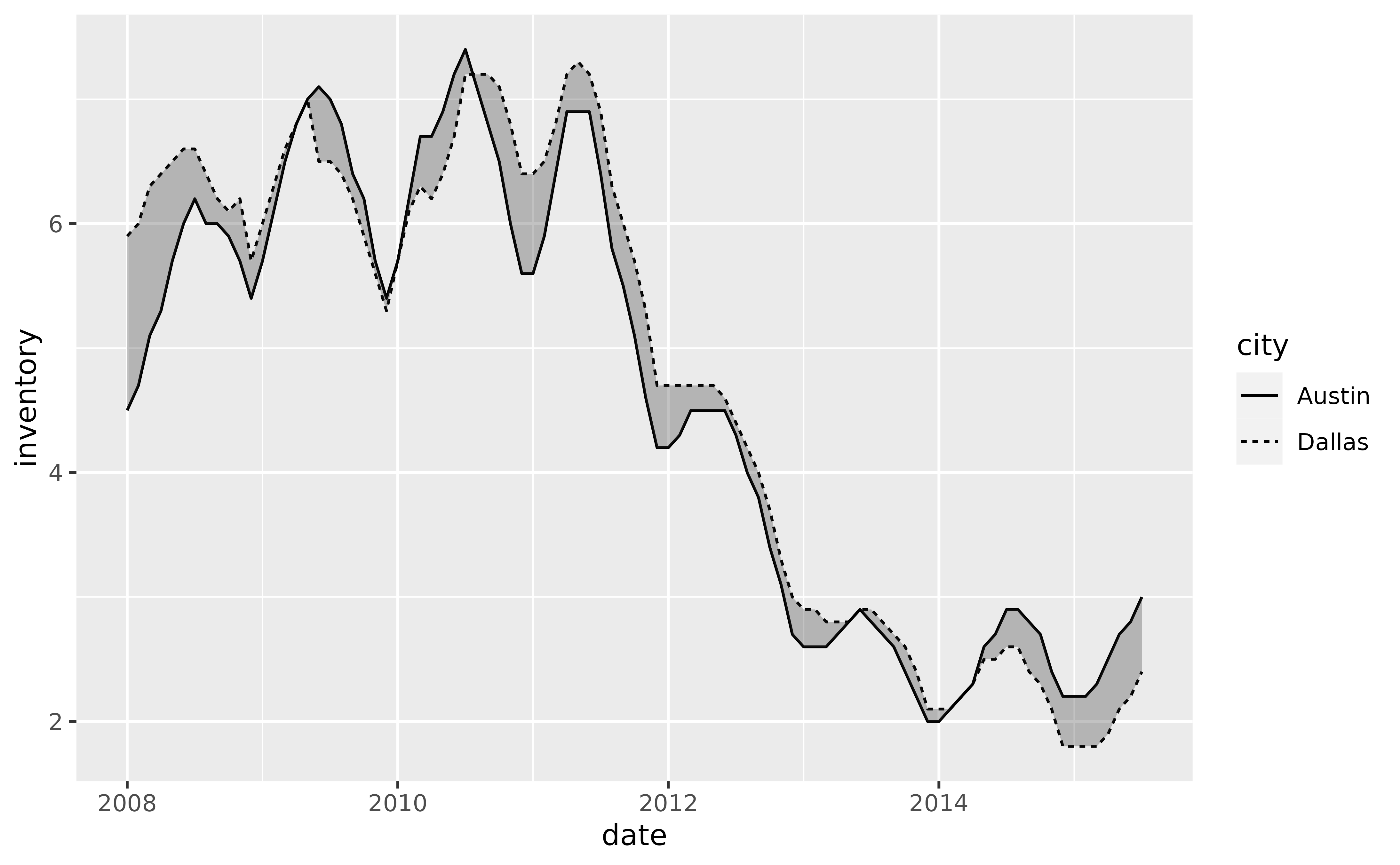# Now fill the ribbon between the two series with different colors depending
# on which series is over or under the other. Do so by mapping any of the
# following to the fill aesthetic:
#   austin < dallas
#   austin > dallas
#   austin <= dallas
#   austin >= dallas
p +
geom_line(aes(y = inventory, linetype = city)) +
geom_braid(
aes(ymin = austin, ymax = dallas, fill = austin > dallas),
data = tx_wide,
method = "line",
alpha = 0.6
)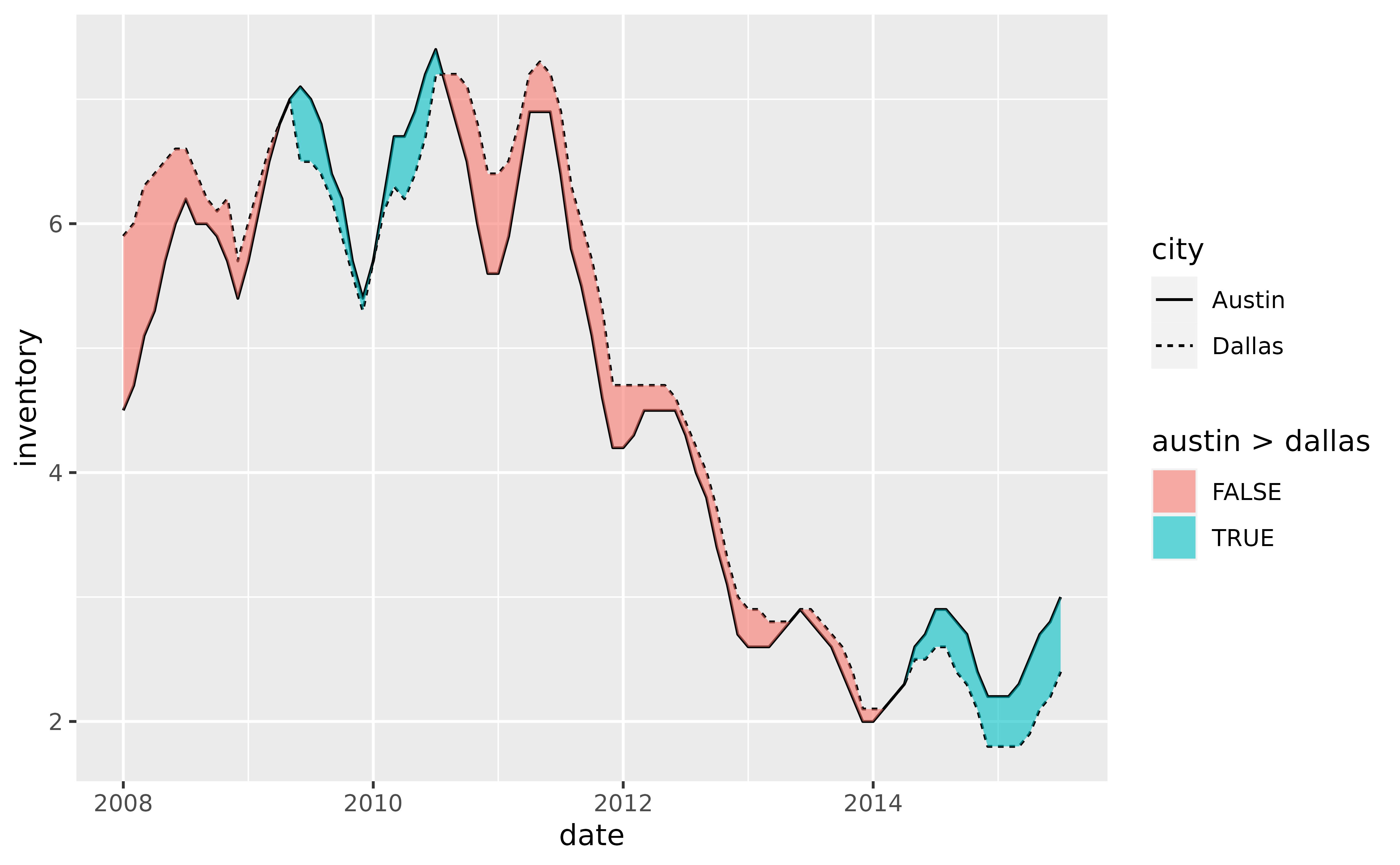# Alternatively, map after_stat(braid) to fill which will apply
# ymin < ymax by default, in this case austin < dallas
p +
geom_line(aes(y = inventory, linetype = city)) +
geom_braid(
aes(ymin = austin, ymax = dallas, fill = after_stat(braid)),
data = tx_wide,
method = "line",
alpha = 0.6
)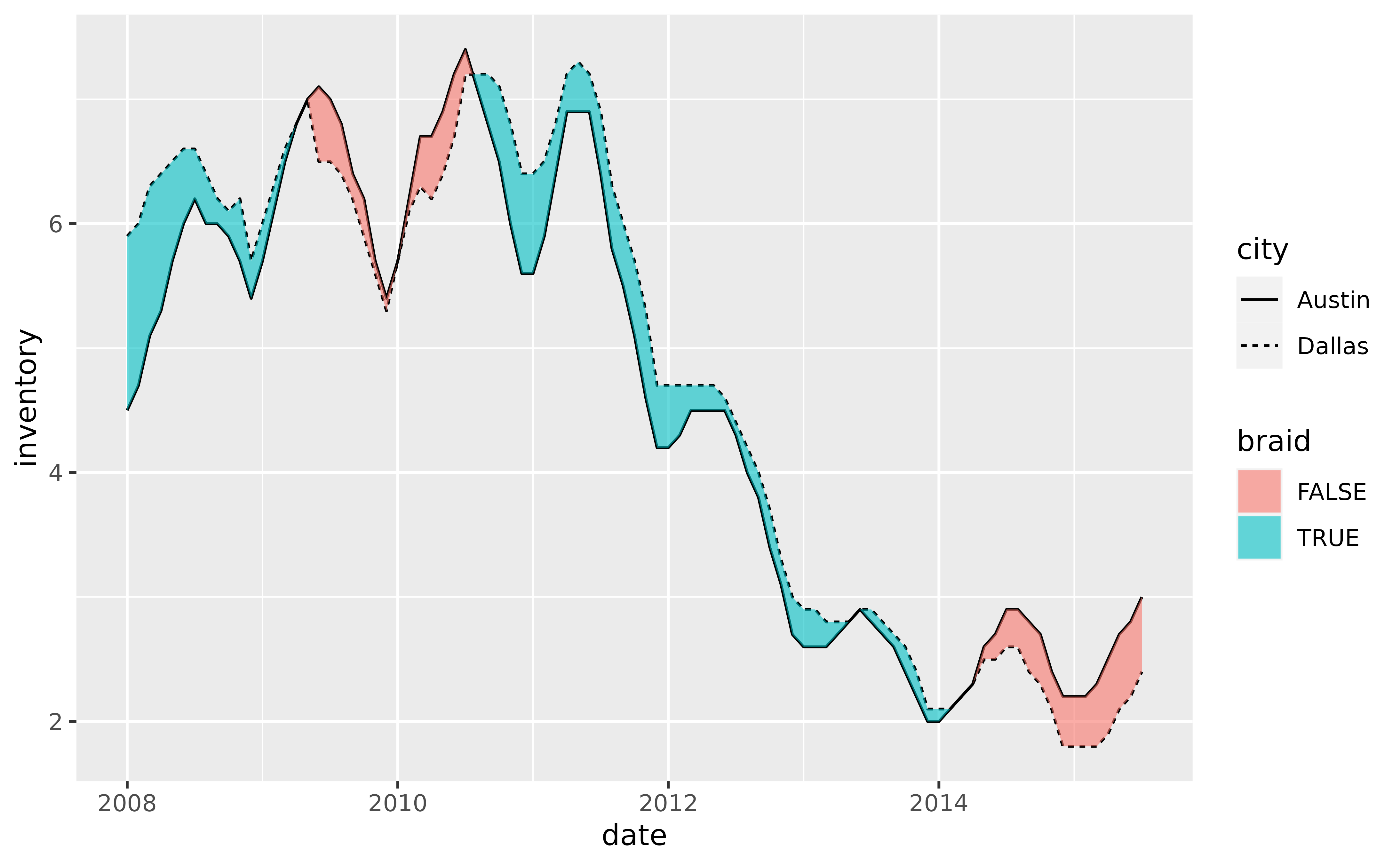# To braid a ribbon with two series drawn with geom_step(), use
# method = "step" in geom_braid().
p +
geom_step(aes(y = inventory, linetype = city)) +
geom_braid(
aes(ymin = austin, ymax = dallas),
data = tx_wide,
method = "step",
alpha = 0.3
)p +
geom_step(aes(y = inventory, linetype = city)) +
geom_braid(
aes(ymin = austin, ymax = dallas, fill = austin < dallas),
data = tx_wide,
method = "step",
alpha = 0.6
)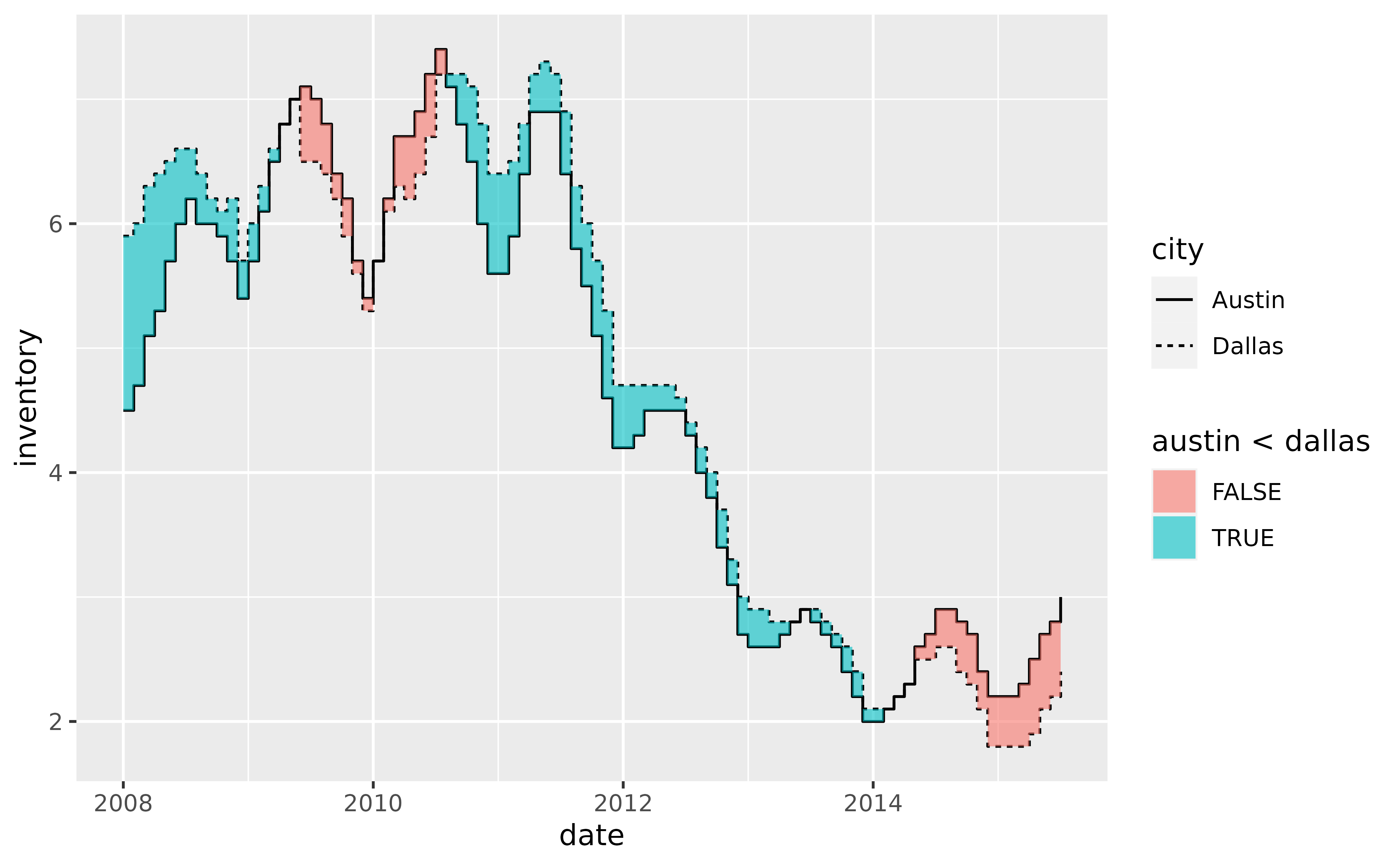# How does geom_braid() handle missing values? Let's replace some existing
# values with NAs to demonstrate.

set.seed(42)  # for reproducibility

tx_long[sample(1:nrow(tx_long), 20), "inventory"] <- NA

tx_wide <- transform(tx_wide,
dallas = with(tx_long, inventory[city == "Dallas"]),
austin = with(tx_long, inventory[city == "Austin"])
)

p <- ggplot(tx_long, aes(date))

p + geom_line(aes(y = inventory, linetype = city), na.rm = TRUE)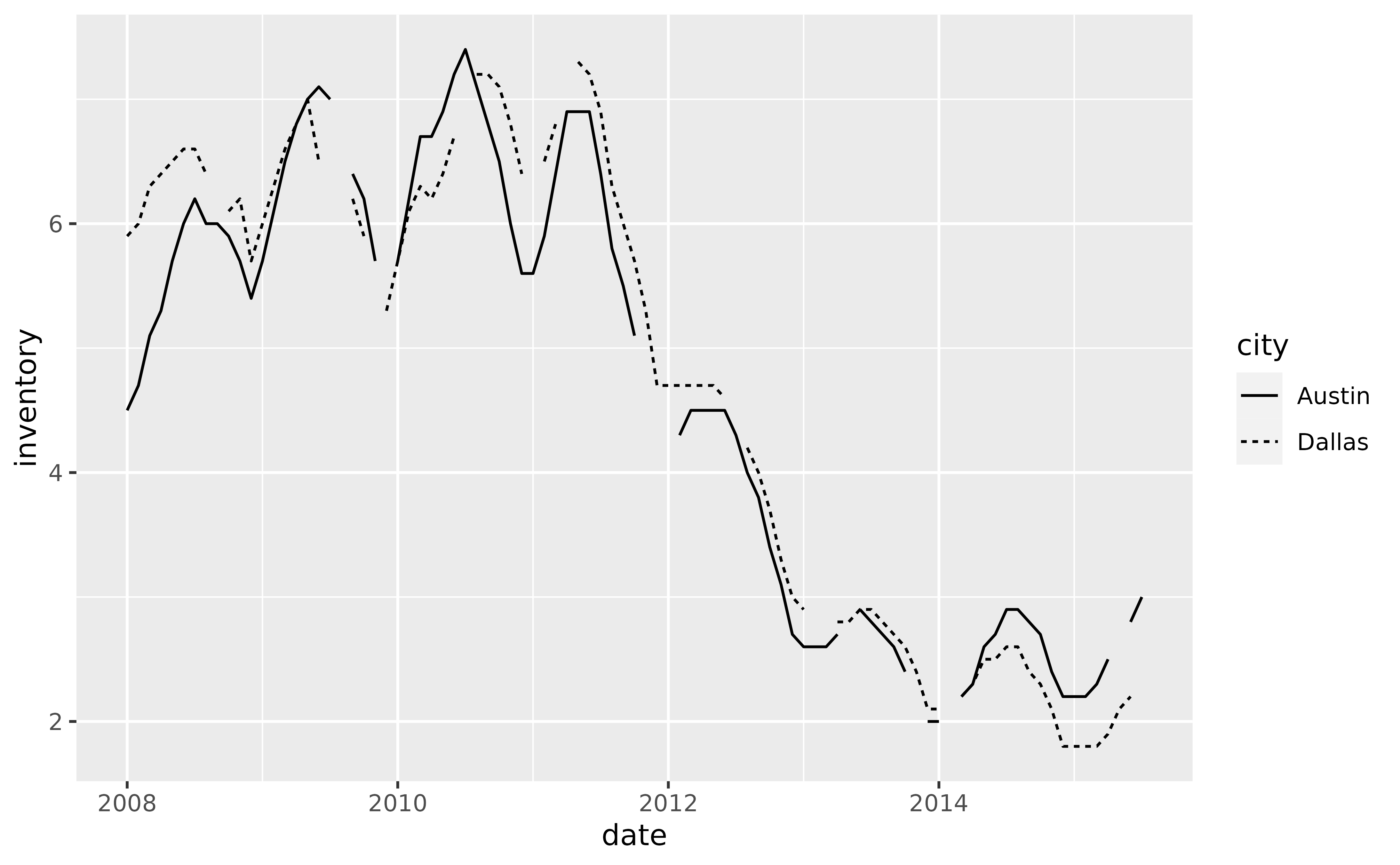# If na.rm = NA, the default, geom_braid() imputes missing values that
# occur between observations in a series.
p +
geom_line(aes(y = inventory, linetype = city), na.rm = TRUE) +
geom_braid(
aes(ymin = austin, ymax = dallas, fill = austin < dallas),
data = tx_wide,
method = "line",
alpha = 0.6
)# If na.rm = FALSE, geom_braid() keeps the missing values and portrays
# them as gaps in the ribbon.
p +
geom_line(aes(y = inventory, linetype = city), na.rm = TRUE) +
geom_braid(
aes(ymin = austin, ymax = dallas, fill = austin < dallas),
data = tx_wide,
method = "line",
alpha = 0.6,
na.rm = FALSE
)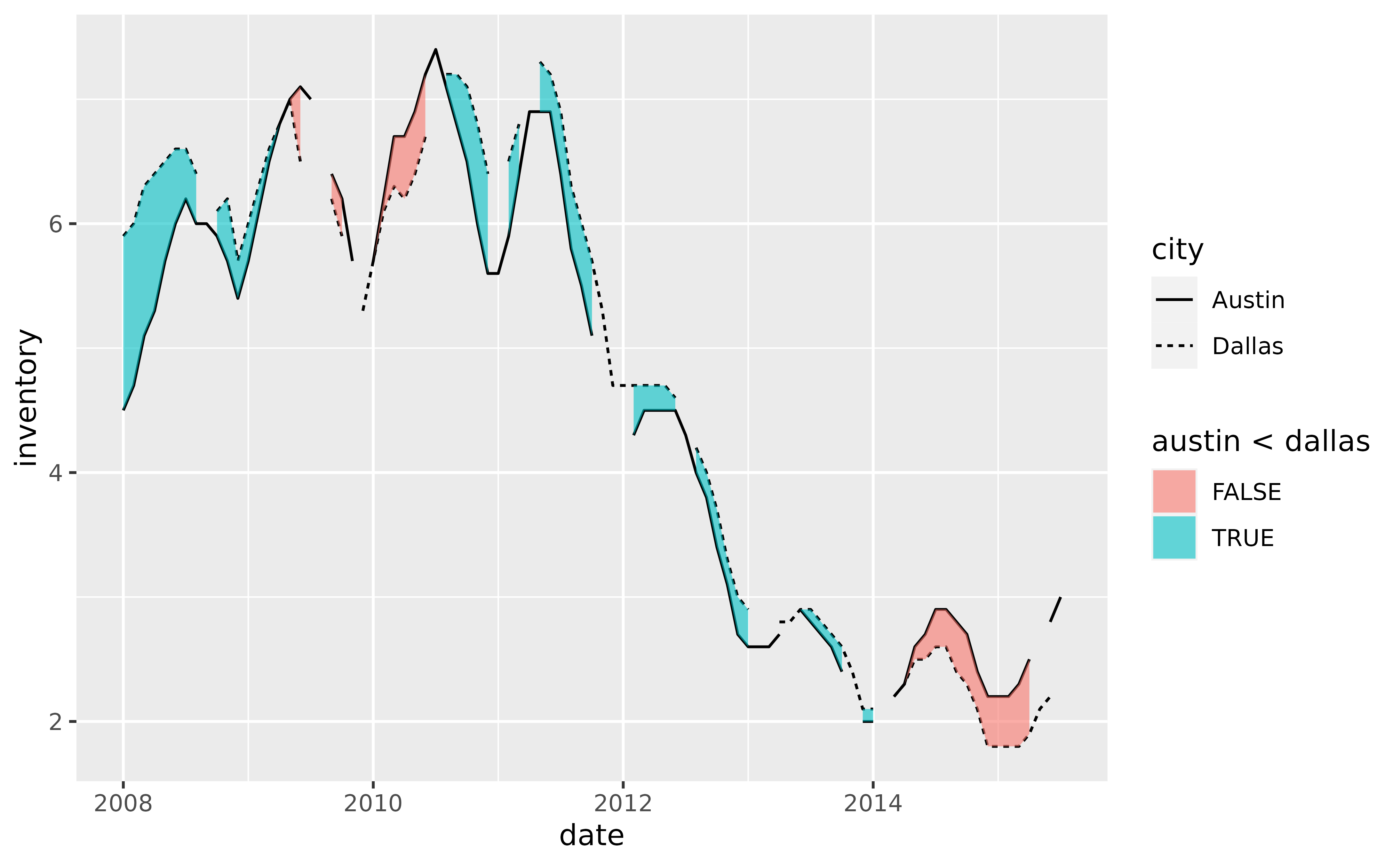# If na.rm = TRUE, geom_braid() removes the missing values. However,
# because this removes rows in tx_wide where only one of austin and
# dallas may be missing, the resulting ribbon will likely not match the
# lines drawn with geom_line() using tx_long.
p +
geom_line(aes(y = inventory, linetype = city), na.rm = TRUE) +
geom_braid(
aes(ymin = austin, ymax = dallas, fill = austin < dallas),
data = tx_wide,
method = "line",
alpha = 0.6,
na.rm = TRUE
)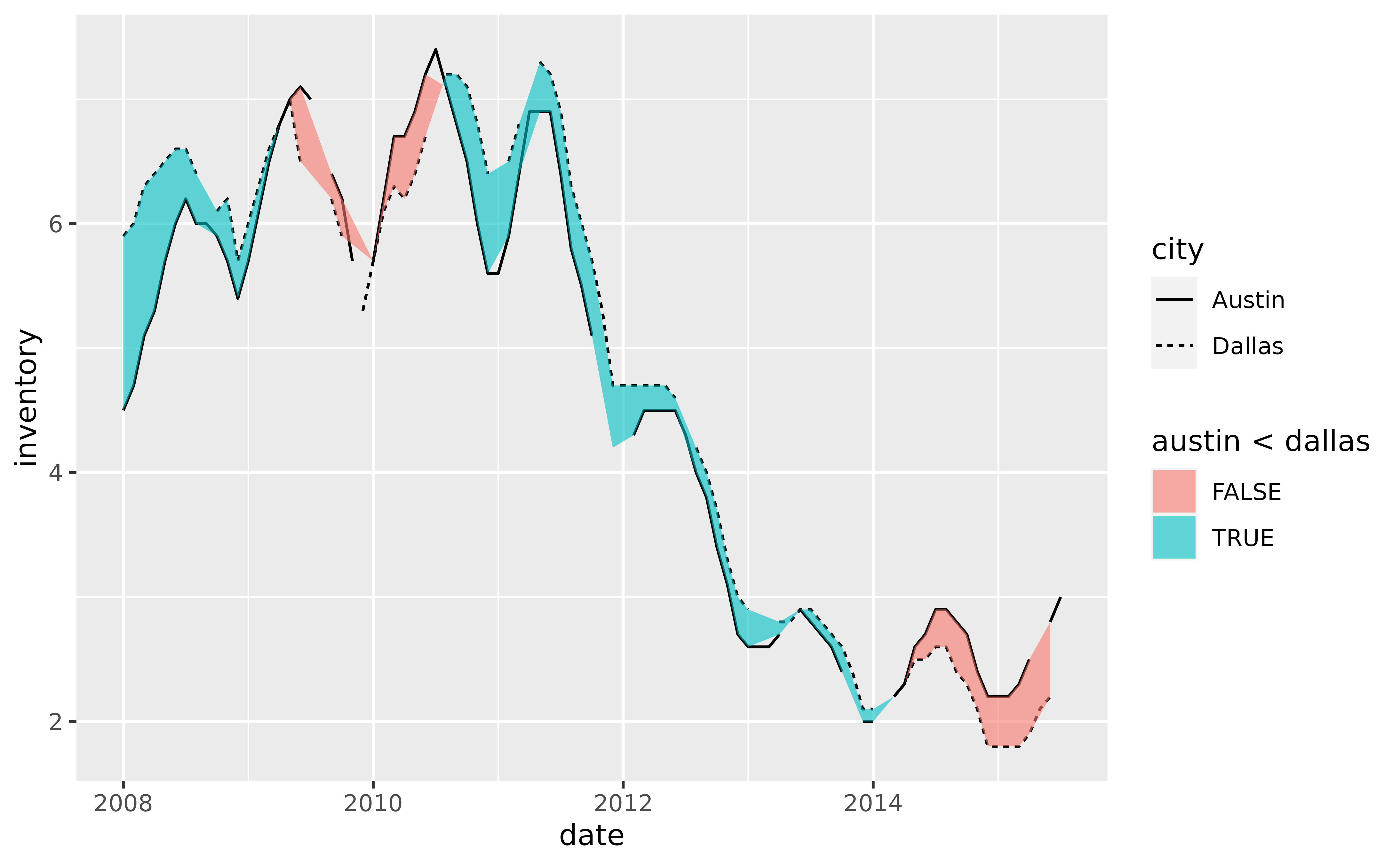# Happy braiding!